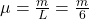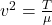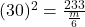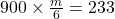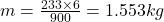## g A rope, under a tension of 233 N and fixed at both ends, oscillates in a second-harmonic standing wave pattern. The displacement of the ro

Question

g A rope, under a tension of 233 N and fixed at both ends, oscillates in a second-harmonic standing wave pattern. The displacement of the rope is given by . where at one end of the rope, is in meters, and is in seconds. What are (a) the length of the rope, (b) the speed of the waves on the rope, and (c) the mass of the rope

in progress 0
6 months 2021-08-02T16:07:39+00:00 1 Answers 7 views 0

We are given that

Tension=T=233 N

The displacement of  the rope is given by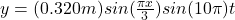a.By comparing with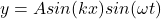We get

A=0.32

k=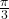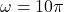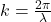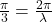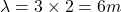n=2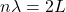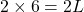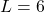m

b.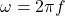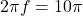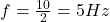Speed,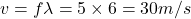c.Let

Mass of the rope=m